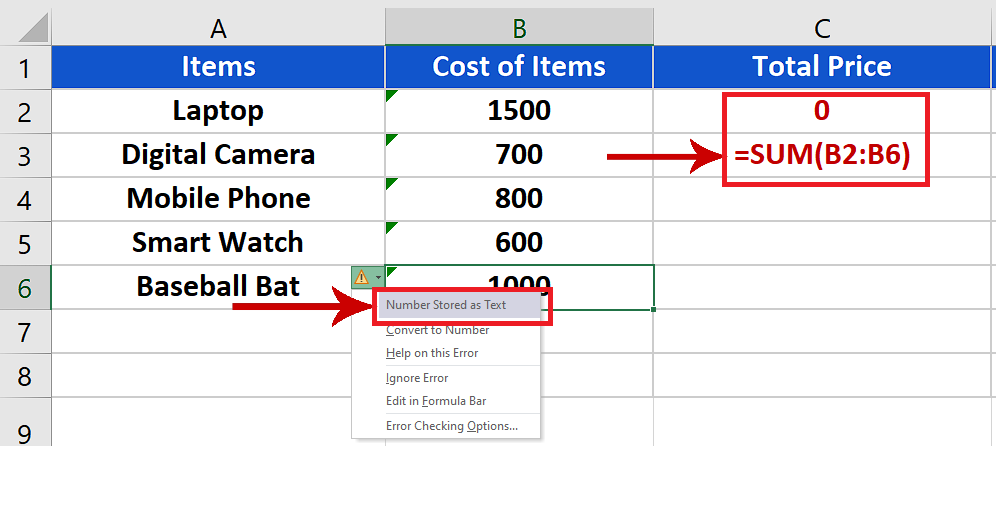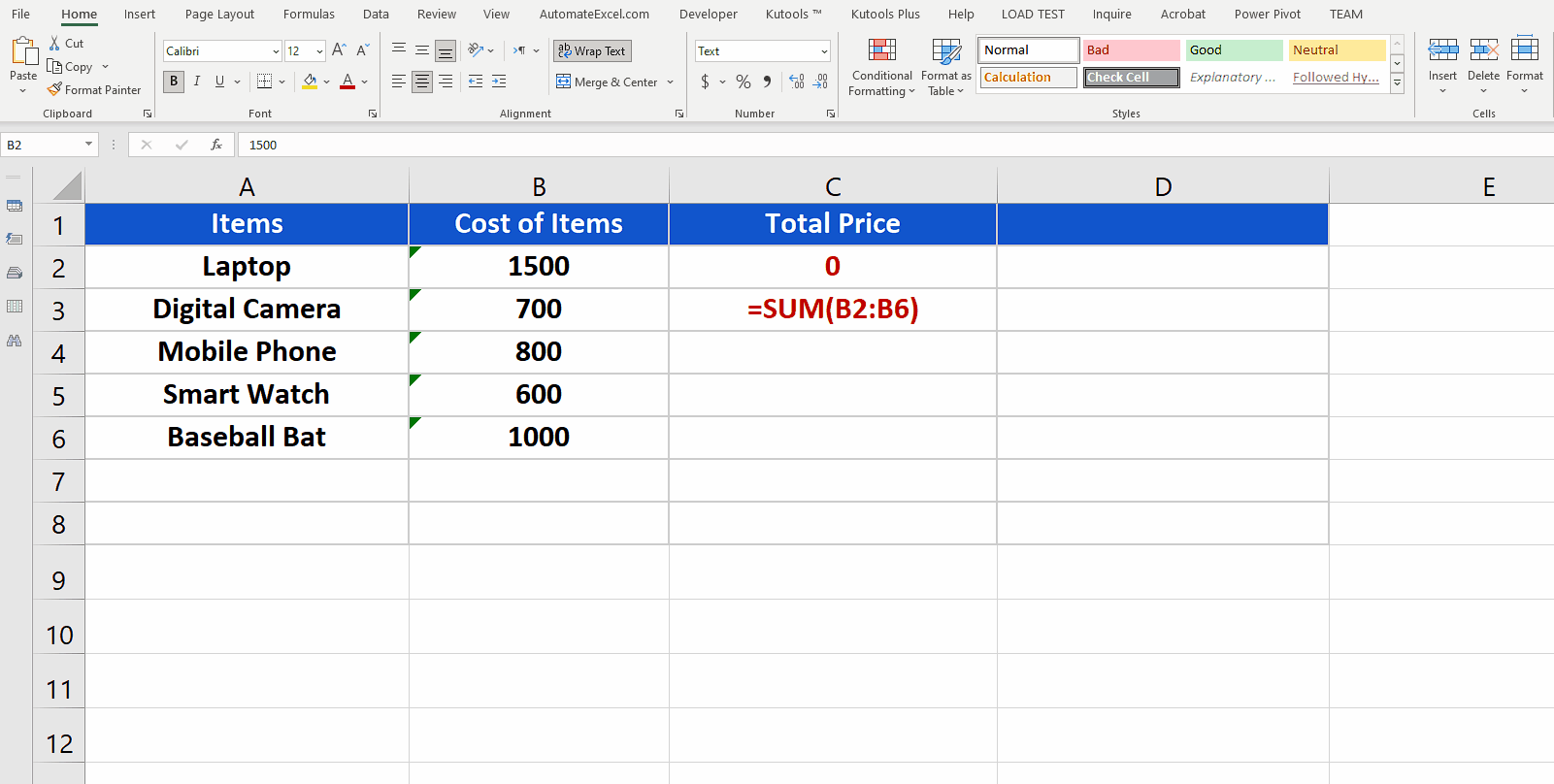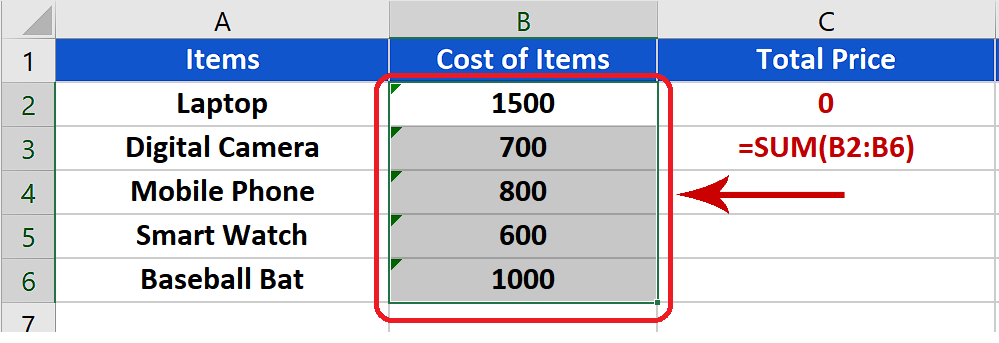# How to fix number stored as Text error in Excel

Sometimes, we wish to get data from somewhere else and show the calculation formulas and the values as well. However, if source data was formatted as text then after pasting those values in your sheet can create a lot of mess for you. Take a look at the example below;We can see this image with a “!” sign which means that there is some problem. When we click on this it will show us the message as shown above. Furthermore, we can see that our total price is showing us a Zero even though we had a formula in place properly to calculate the sum of numbers from B2:B6. To resolve this issue Excel provides us with a simple but tricky method. Let’s learn it step by step.Excel is mainly a data manipulation and analysis tool which provides us with tons of formulas and functions to perform basic mathematical and statistical data analysis. However, at the same time it provides us the ability to manipulate text as well.

### Step 1 – Choose the data cells formatted as text in one column– Select all that data which is formatted as text and is available in one column.

### Step 2 – Convert cells formatted as text back to Numbers– After selecting the desired data, go to the Data tab on the main list of tabs and then locate Text to Columns in the Data Tools group.

– Now press Text to Columns and then press Finish.

– The data will be converted to numbers and the formula will automatically update to show us the correct results.

So this is how we can convert the numbers stored as text back to numbers again.